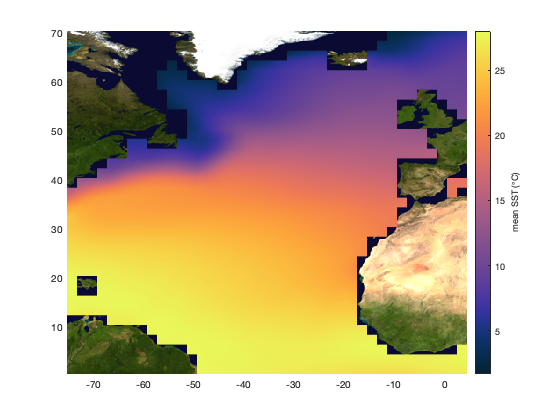# amo documentation

amo computes a version of the Atlantic Multidecadal Oscillation index.

Back to Climate Data Tools Contents

## Syntax

```idx = amo(sst,t)
idx = amo(sst,t,lat,lon)```

## Description

idx = amo(sst,t) calculates AMO index from a time series of sea surface temperatures sst and their corresponding times t. sst can be a vector of sea surface temperatures that have been averaged over a region of interest, or sst can be a 3D matrix whose third dimension correponds to times t. If sst is a 3D matrix, a time series is automatically generated by averaging all the grid cells in sst for each time step. Usually, the AMO is calculated from the area-weighted average of ssts in the northern Atlantic from 0-70ºN.

idx = amo(sst,t,lat,lon) calculates the AMO index for 3D sst time series and corresponding grid coordinates lat,|lon|. Using this syntax, grid cells within the AMO region are automatically determined and the AMO index is calculated from the area-averaged time series of ssts within that region.

## Example

In this example, we will calculate the AMO index using the monthly north_atlantic_sst.mat dataset that comes with CDT. Start by loading the data that comes with CDT. It contains monthly sea surface temperatures from 1870-2017 for the Northern Atlantic region (0-70N)

```load north_atlantic_sst.mat
```

Here are the variables we're working with:

```whos % displays variable names and size
```
```  Name           Size                    Bytes  Class       Attributes

lat           36x1                       144  single
lon           41x1                       164  single
sst_na        36x41x1776            10485504  single
t           1776x1                     28417  datetime

```

The table above tells us that we have a lat array, a lon array, and a t array, which correspond to the dimensions of an sst_na data cube of SSTs in the North Atlantic.

We'll need to tell the amo function exactly what latitudes and longitudes correspond to each grid cell in the sst_na data, so convert the lat and lon arrays into grids like this:

```[Lon,Lat] = meshgrid(lon,lat);
```

To get a little bit of context, let's take a look at the data we'll be working with. Make a pcolor plot of time-averaged mean SSTs (down dimension 3 of the sst_na data cube). Set the colormap to thermal via the cmocean function, and underlay with an earthimage to give a better sense of where we're looking at:

```pcolor(Lon,Lat,mean(sst_na,3))
cmocean thermal % sets the colormap
cb = colorbar;
ylabel(cb,'mean SST (\circC)')
hold on
earthimage('bottom') % underlay with a satellite image of Earth
```The map above shows only the region from 0N to 70N and 75W to 5 E. That's the geoquad the amo function uses to calculate the AMO index, but if you're working with an SST dataset that covers a larger area, don't worry--the amo function uses the Lat,Lon grids you give it to make calculations based only on values in the North Atlantic.

## Calculate the AMO Index

The amo function works by entering the sst data cube along with corresponding times and Lat,Lon grids:

```idx = amo(sst_na,t,Lat,Lon);
```

Plot the raw AMO index:

```figure
plot(t,idx)
ylabel 'AMO index'
```The plot above shows North Atlantic sea surface temperature variability, and it seems to have a sort of oscillatory behavior, and the periods of the oscillations appears to be multiple decades long. You might say it's some sort of Atlantic Multidecadal Oscillation.

NOAA recommends taking a 10 year (121 month) moving average to smooth out the time series:

```% Low-pass filter with a 121 month moving average:
idx_f = movmean(idx,121);

hold on
plot(t,idx_f,'k-','linewidth',2)
legend('AMO index','AMO (10 year moving mean)')
```To show we're on the right track, we can use the anomaly function to mimic Figure 1a from Enfield et al., 2001:

```figure
anomaly(datenum(t),idx_f,...
'topcolor',rgb('orange'),'bottomcolor',rgb('yellow'))
axis tight
datetick('x')
xlim(datenum([1860 2000],1,1)) % sets x axis date limits
ylim([-0.3 0.3])               % sets y axis limits
```Figure 1b of Enfield et al. shows the correlation between the AMO index and global sea surface temperatures. Unfortunately, our sample dataset only covers the North Atlantic, so for a comparison to other places around the globe we'll have to use the pacific_sst sample dataset:

```P = load('pacific_sst.mat');
```

Enfield et al. correlated the lowpass-filtered AMO (which is inherently deseasoned) to deseasoned, lowpass-filtered global SSTs. So let's deseason and lowpass filter the Pacific Ocean SST dataset:

```% Deseason and lowpass filter SSTs:
sstd = deseason(P.sst,P.t);
sstdf = movmean(sstd,121,3);
```

Before we can correlate our lowpass-filtered AMO index to the deseasoned, lowpass-filtered Pacific SSTs, we'll have to synchronize the two time series. An easy way to do that is to interpolate the idx_f array to the Pacific SST times, like this:

```idx_fi = interp1(datenum(t),idx_f,P.t);

% Plot the interpolated values to ensure everything lines up:
hold on
plot(P.t,idx_fi,'b-','linewidth',4)
```The fat blue line above indicates we've correctly interpolated the idx_f values to the times P.t, which correspond to the times of the Pacific Ocean SST time series.

Now we're able to correlate the lowpass-filtered, interpolated AMO index idx_fi with Pacific SSTs:

```% Correlate ssts to AMO:
C = corr3(sstdf,idx_fi);

figure
imagescn(P.lon,P.lat,C)
caxis([-1 1])
cmocean balance
```The map above does not exactly match figure 1b from Enfield et al., likely because we're only comparing the AMO to 802 months (66 years) of a multi-decadal phenomenon. A proper correlation would look at several complete cycles of the oscillation.

## Reference

Enfield, D.B., A.M. Mestas-Nunez, and P.J. Trimble, 2001: The Atlantic Multidecadal Oscillation and its relationship to rainfall and river flows in the continental U.S., Geophys. Res. Lett., 28: 2077-2080. doi:0.1029/2000GL012745

## Author Info

This function was written by Kaustubh Thirumalai of the University of Arizona, for the Climate Data Toolbox for Matlab. March 2019. http://www.kaustubh.info